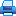Address: office 1410a, 17 Naberezhnaya Severnoy Dviny, Arkhangelsk, 163002, Russian Federation, Northern (Arctic) Federal University named after M.V. Lomonosov

Phone: (818-2) 21-61-21
E-mail: vestnik_est@narfu.ru
http://aer.narfu.ru/en/

# The Number Decompositions of Matrices in the Subgroup of Rc. P. 115–121Section: Physics. Mathematics. Informatics

512.541+512.542

### Authors

Popov Ivan Nikolaevich
Institute of Mathematics, Information and Space Technologies, Northern (Arctic) Federal University named after M.V. Lomonosov (Arkhangelsk, Russia)
e-mail: only-for-you-pi@mail.ru

### Abstract

The results of the researches on the group RCn are outlined in the paper. The elements of the group RC are dimension n square matrices over the field Z2, the group operation is the addition of matrices. Any matrix in the finite additive group RC has order 2 and it can be represented as a sum of the so-called row-matrices or column-matrices. The range of the row-matrices and the column-matrices generates a carrier of the group element. Every matrix from RC has exactly two different carriers. Every subgroup in RC can be considered as a linear span of matrices, which is called a “generator span’. The span can have several sets of generators. Among all forming linear span there are the socalled fundamental systems, through each any span element is expressed in a unique way. This paper examines the question of the number decompositions of matrices in the subgroup of RC defined as a linear span with a certain number of generators. A relation between a number of decompositions of arbitrary matrices with a number of expansions of zero matrix in terms of the generators of the linear span. A theorem which asserts that the number decompositions of the matrix in the subgroup of RC is equal to the number of expansions of zero matrix in this subgroup is formulated and proved. A formula to determine the number of all decompositions of zero matrix in an arbitrary subgroup of the RC is proposed. The article deals with the issue of a fundamental system of subgroup generators of RC and its role in determining the order of the linear span as a subgroup of RC and the group RC.

### Keywords

the order of the group, linear span, fundamental system of generators